## Why is this example from Maple not working?...

F := proc(t)
plottools[line]([-2,0], [cos(t)-2, sin(t)], color=blue),
plottools[line]([cos(t)-2, sin(t)], [t, sin(t)], color=blue),
plot(sin(x), x=0..t, view=[-3..7, -5..5]);
end proc:

animate(F,[theta],theta=0..2*Pi,background=plot([cos(t)-2,sin(t),t=0..2*Pi]),
scaling=constrained,axes=none);

Why is it not working?

Thank you!

mapleatha

## Tensor summation...

Hi, I have a problem with tensor calculus. Please guide me.

I have two Tensors B[mu, nu] and A[~mu, ~nu].

Furthermore, I have two mixed tensors as U[~1, mu, nu] and U[~2,mu,nu]

rho, mu, and nu can run from 1 to 2. Hence, A, B, and both Us are 2*2 matrices.

Note that there was only one U, but since it is a mixed tensor, hence I have separated it into two tensors for making it easy.

Now I want to compute the following summation:

U[~rho,mu,nu] U[~alpha,beta,gamma] B[rho,alpha] A[~mu,~beta] A[~nu,~gamma].

Maple must give a number for rho, mu, nu, alpha, beta, and gamma (which can take 1 and 2) and compute "all" possible summations. Since the result will be just "a number", hence I could not use TensorArray.

How can I do it and get a result?

The forms of all tensors are in the attached maple file:
question.mw

## how to change subexpression with some specific pat...

I need to change any occurance of   anything*sqrt(anything)  to say Z in any large expression.  (later, I can add the correct replacement once I know how to do it for Z).

I can change   sqrt(anything) with the help of the answers in Substitution-Of-Sub-Expressions-Is  but not  anything*sqrt(anything)

Here is an example to make it clear. Given

`expr:=(-x + sqrt(9*x^2*exp(2*c) + 8)*exp(-c)+99)/(4*x)+ (a*sqrt(z)-99+sin(c*sqrt(r+4)+20))/3+10+1/(c+exp(-x)*sqrt(exp(x)))+sqrt(h)`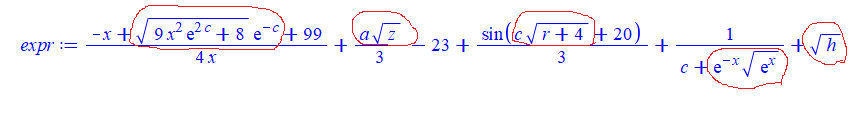Need to all some transformation on  those subexpressions circled above. For now, lets say I wanted to replace them with so it should becomes this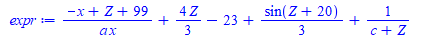I can do this

`subsindets(expr,anything^({1/2,-1/2}),ee->Z)`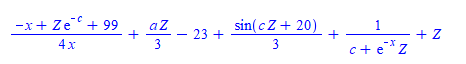But I need to have the term (if any) that multiplies the sqrt as well included.

And that is the problem. Can't figure how to do it. When I try

`subsindets(expr,anything*anything^({1/2,-1/2}),ee->Z)`

Maple says Error, testing against an invalid type Ok. So anything*anything^({1/2,-1/2}) is not a type. What to do then?  Tried also subsindets(expr,t::anything*anything^({1/2,-1/2}),ee->Z) same error

And

```applyrule(t1::anything*sqrt(t0::anything)=Z, expr);
```

gives  which is wrong.

How to make this work in Maple using subsindets? Can one use pattern with subsindets? How to make a type for

anything*sqrt(anything)

Maple 2021.1

## Extract specific items of expression....

I 'd like to extract all items that contain "a" in an expression except "ab". What I can think of is to use the two functions select and remove to do it, but I feel a little less automated. Is there a better way?

f := a*b+a*c+a+b+c
s:=remove(has,select(has,[op(f)],a),a*b)

s := [a c, a]

## differentiation methods tutor answer syntax questi...

Hi,

I'm a student learning calculus I

Would someone explain what the synax in the steps means? I don't understand what is going on with the section that has _X0 in it.## How to suppress axes labels...

I want to plot a 2D graph without labels. The labes=["",""] option does half of the job—it prints the empty string for labels (that's good) but it reserves room for them (that's bad).  In the following code I use a large size labelfont in order to exaggerate the effect:

```restart;
plots:-setoptions(labelfont=[TIMES,64]);  # large labelfont selected on purpose
p1 := plot([[0,0],[1,1]], labels=["", ""]);```Note the large blank space at the bottom reserved for the the non-existent label.

I know one way to eliminate the label altogether:

`p2 := subs(AXESLABELS=NULL, p1);`This does the right job but is there a more orthodox way of doing that?

Afterthought:  It would be good if the labels option to the plot command  accepted none as argument, as in labels=[none, none].

## on output format from solve with exponential...

Why Maple likes to extract exp() outside the sqrt when its argument has minus sign vs. not?  Compare the following

```restart;
eq2 := ln(2*u^2 + u - 1) = -c - 2*ln(x);
sol:=[solve(eq2,u)]:
simplify(sol)
```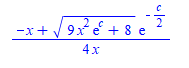and

```eq2 := ln(2*u^2 + u - 1) = c - 2*ln(x);
sol:=[solve(eq2,u)]:
simplify(sol)
```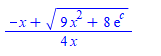I like the above much better than the first one. Mathematica keeps both same form (i.e. keeps the exp() inside):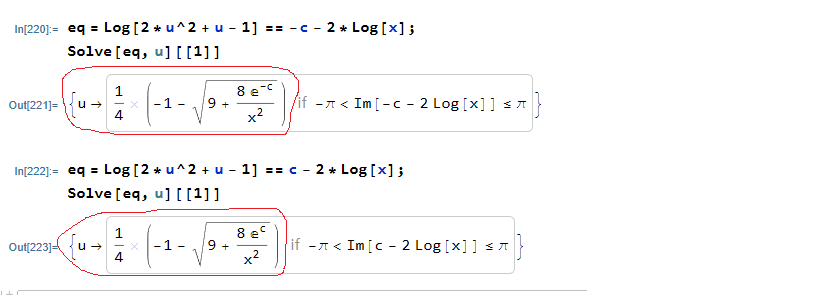Maple's answers are correct ofcourse, I just do not understand the logic why when there is a minus sign on it likes to format it differently as shown.

Is there a way to make not do that?

## Getting the correct derivative of a power in a Sum...

I am trying to get Maple to recognize that

diff(x^n,x) does not equal n*x^(n-1), but rather 0 if n=0, or n*x^(n-1) otherwise.

This comes up when differentiating an infinite sum (power series). The constant term gets transformed into n/x instead of becoming zero. Maybe this is really a bug/lack of feature in how sum/Sum works.

For example: diff(Sum(x^n, n = 0 .. infinity), x) yields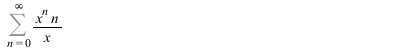Does anybody have a fix to get the differentiation right (other than expanding some terms of the series before taking the derivative)?

(edited) Please note I am not trying to get an answer to this specific question. It is just illustrates a simplified example of behavior that leads to other bizarre results. Please see the attached worksheet for more weirdness: Problems_with_summation_Mapleprimes.mw

## how can i remove, zero after dot from my result...

how can i remove, zero after dot from my result

restart;
V := 4;
K := 16.56;

H := 0.5;

q1 := evalf(diff(y(x), x) = V*y(x)/H - K*y(x)/H);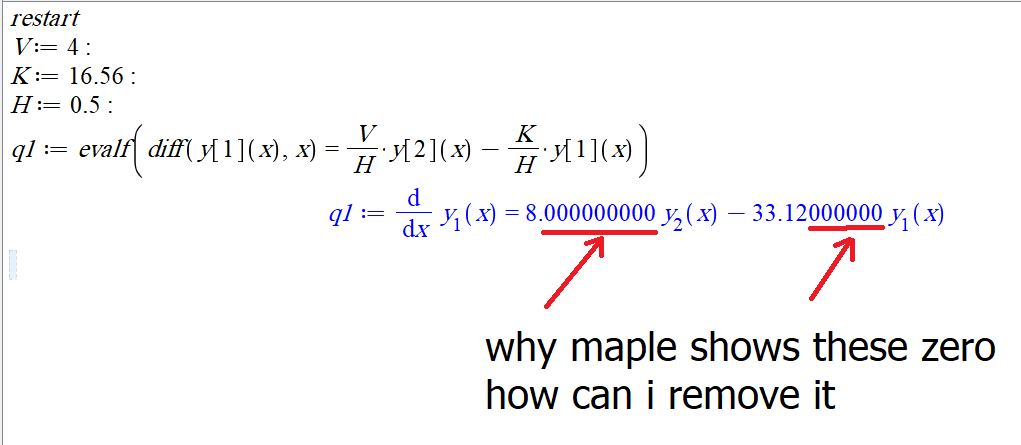## How to find the Jacobian in this case? ...

Hello

Consider

```alias(x=x(t),y=y(t),phi1=phi1(x(t),y(t)));

phi:=[x,ph1];```

I need to calculate the Jacobian of phi in relation to x and y (and finally to t)  but using VectorCalculus[Jacobian] does not work. I guess it is because of the time dependence.   What am I missing?

Can the Physics package be used?

Many thanks.

## Iterate with alphabets in maple...

Hello,
I want to update Alphabets in my ‘for loop’ instead of numbers. Please look at the code below:

for Tl from 263 by 5 to 313 do
for Ql from 25 by 25 to 300 do
myvel:= my_porous(Ql, Tl, ratiol);
Export(<myvel>, "HPporousmodel.xlsx", 1, my_alph[vcount]);
vcount := vcount+1;

end do

end do

Here, my_alph is a manually created alphabets corresponding to excel columns as below:

my_alph:=[ "A1", "B1", "C1", "D1", "E1", "F1", "G1", "H1", "I1", "J1", "K1", "L1", "M1", "N1", "O1", "P1", "Q1", "R1", "S1", "T1", "U1", "V1", "W1", "X1", "Y1", "Z1", "AA1", "AB1", "AC1", "AD1", "AE1", "AF1", "AG1", "AH1", "AI1", "AJ1", "AK1", "AL1", "AM1", "AN1", "AO1", "AP1", "AQ1", "AR1", "AS1", "AT1", "AU1", "AV1", "AW1", "AX1", "AY1", "AZ1", "BA1", "BB1", "BC1", "BD1", "BE1", "BF1", "BG1", "BH1", "BI1", "BJ1", "BK1", "BL1", "BM1", "BN1", "BO1", "BP1", "BQ1", "BR1", "BS1", "BT1", "BU1", "BV1", "BW1", "BX1", "BY1", "BZ1"]:

The idea is to export the velocity vector that I get from proc (my_porous(Ql, Tl, ratiol)) in to an excel sheet (HPporousmodel.xlsx)with the command (Export(<myvel>, "HPporousmodel.xlsx", 1, my_alph[vcount]);). I want to export the velocities into a new column for every iteration starting from A, B, C,….. and so on. However, since I don’t know how to do that I’ve used a manually created list my_alph. This has a big disadvantage of restricting the number of times I can loop. For this case, I can only loop (3*26=78 times). Anything more asks for manually increasing the entries of my my_alph list. I want to loop for 100's of times which is becoming a big problem with this method.

Can anyone please help me in automating this without any such constraints as we do with numbers (like we have done for vcount here)?

Thank you.

## Exporting 3D Plot in Vector Graphics Format...

I am trying to export a 3D plot from Maple (2021) in a vector format that can be imported into Adobe Illustrator without too much trouble. The graphic artist says the PDF files are not useful (“at the base of every PDF is an image file, inside layers and layers of clipping paths; not helpful”). “SVG does not appear to produce usable vectors – well, maybe not, but the one you sent me was super difficult to wrangle”. She had hoped that EPS would be useful, but she was unable to open the EPS file I created (not enough memory).

Here is the example that I have been using as I've asked others for help with this question.

```restart;
with(plots);
with(plottools);

T := torus([0, 0, 0], 1/2, 1/2);
C0 := spacecurve([cos(t), sin(t), 0], t = 0 .. 2*Pi, color = red, thickness = 9);
C1 := spacecurve([0.5*cos(t), 0.5*sin(t), 0.5], t = 0 .. 2*Pi, color = red, thickness = 9);
C2 := spacecurve([0.5*(1 + cos(t)), 0, 0.5*sin(t)], t = 0 .. Pi, color = blue, thickness = 9);
C3 := spacecurve([0.5*(-1 + cos(t)), 0, 0.5*sin(t)], t = 0 .. Pi, color = blue, thickness = 9);
Fig9510 := display([T, C0, C1, C2], view = [DEFAULT, DEFAULT, 0 .. 1/2], scaling = constrained, transparency = 0.75);```

Direct links to the worksheet and the PDF, EPS, and SVG files on DropBox are provide below in the hope that something will be useful to somebody. (MaplePrimes links are provided for the MW and PDF files are also provided - but EPS and SVG files are not permitted.)

1. 3DPlotExample.mw: https://www.dropbox.com/s/mx1hg1ntuqihnu3/3DPlotExample.mw?dl=0
2. Fig9510.pdf: https://www.dropbox.com/s/bnzfqrh060gw84g/Fig9510.pdf?dl=0
3. Fig9510.eps: https://www.dropbox.com/s/h9bjyn0uwm8w5ct/Fig9510.eps?dl=0
4. Fig9510.svg: https://www.dropbox.com/s/u2xfntnqvbhu810/Fig9510.svg?dl=0

This question has been asked for more than ten years. The best solution to has been to use the command-line-interface version of Maple.

1. Is the command-line interface still the best/only option for vector-based graphics output?
2. If so, how do i access the command-line version of Maple 2021 on MacOS?

## Problem with maple ...

Hello, I have a program which works fine in maple but get stuck when I use it in maple 2021 please I need your help thank you in advance

mpprime.mw

Hi all,

This is my first time working on a problem using Maple, and I've run into some issues regarding plotting my findings. After putting in the information for the plot (p:= plot([y1], t = 0..56562.16837, y = 0.10]), I keep receiving the warning "Expecting only range variable t in expression y to be plotted but found name y. I attached the Maple worksheet below for more information, trying to work my way through plotting in Maple.

Worksheet.mw

## How to have a nice display of piecewise in this co...

Hi,

I would like the display of a piecewise-function F with DocumentTools:-Tabulate to look like the one I get with print.
How is it possible to do this?

Here is an example

```restart:
with(DocumentTools):
with(DocumentTools:-Layout):

MV := kernelopts(version);
Maple 2015.2, APPLE UNIVERSAL OSX, Dec 20 2015, Build ID 1097895

# Extract the version number for subsequent use
MV := parse(substring(StringTools:-Split(convert(MV, string), ","), 7..-1))

# I want to display F and G while using DocumentTools
# As you see the display of F is not very pretty.
# This is not a problem related to the "height" of the formula for G is pretty-displayed
# even if it as roughly the same "height" than F

F := piecewise(seq(op([x<k, k]), k=1..5)):
g := x -> 1/(1+x):
G := (g@@5)(x):
Tabulate([F, G]):

# using DocumentTools:-Layout doesn't help

C1 := Cell( Textfield(style=TwoDimOutput,Equation(F))):
C2 := Cell( Textfield(style=TwoDimOutput,Equation(G))):
T  := Table(Column(),Column(),
Row( C1, C2 )
):

if MV < 2018 then
InsertContent(Worksheet(Group(Input( T )))):
else
InsertContent(Worksheet( T )):
end if;
```

This is what DocumentTools:-Tabulate displaysDocumentTools_piecewise.mw

TIA

﻿